# HBSE 6th Class Maths Solutions Chapter 9 Data Handling Ex 9.1

Haryana State Board HBSE 6th Class Maths Solutions Chapter 9 Data Handling Ex 9.1 Textbook Exercise Questions and Answers.

## Haryana Board 6th Class Maths Solutions Chapter 9 Data Handling Exercise 9.1

Question 1.
In a mathematics test, the following marks were obtained by 40 students. Arrange these marks in a table using tally marks.
(a) Find how many students obtained marks equal to or more than 7 ?
(b) How many students obtained marks below 4 ?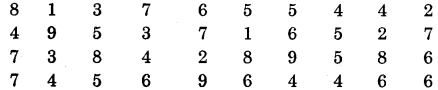Solution: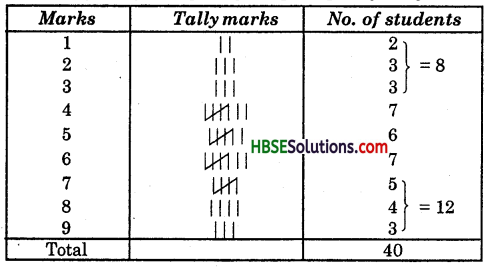(a) 12 students obtained marks equal to or more than 7.
(b) 8 students obtained marks below 4.

Question 2.
Following is the choice of sweets of 30 students of class VI.
(a) Arrange the names of sweets in a table using tally marks.
(b) Which sweet is preferred by most of the students ?
(c) Choose any symbol representing one student and make apictograph showing the choices.
Solution: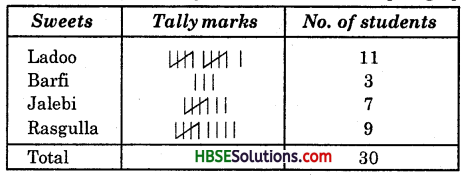(b) Ladoo is preferred by most of the students.
(c) Pictograph.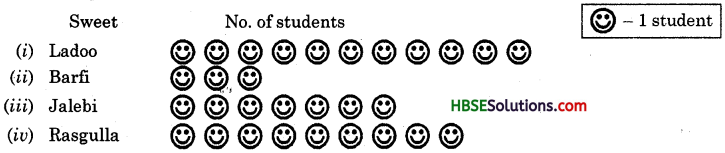Question 3.
Following pictograph shows the number of tractors in 5 villages :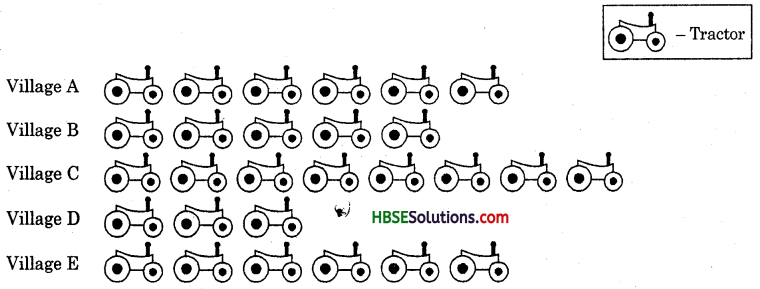Observe the pictograph and answer the following questions :
(i) Which village has the minimum number of tractors ?
(ii) Which village has the maximum number of tractors ?
(iii) How many more tractors village C has as compared to village B ?
(iv) What is the total number of tractors in all the five villages ?
Solution:
(i) Village D has minimum number of tractors.
(ii) Village C has maximum number of tractors.
(iii) Village C has 3 more tractors as compared to village B.
(iv) 6 + 5 + 8 + 3 + 6 = 28. The total number of tractors in all the five villages = 28.

Question 4.
The sale of electric bulbs on different days of a week is shown below :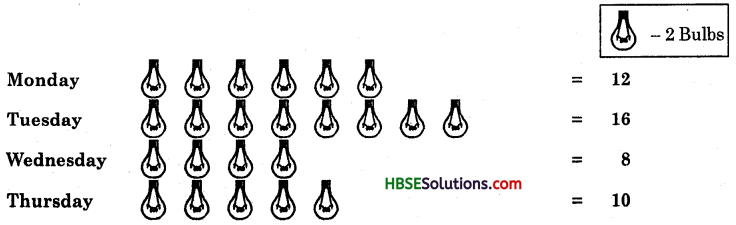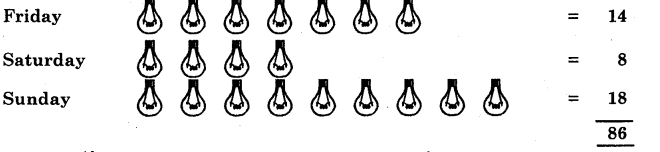Observe the pictograph and answer the following questions :
(a) How many bulbs were sold on Friday ?
(b) On which day were the maximum num ber of bulbs sold ?
(c) If one bulb were sold at the rate ofRs. 10, what was the total sale on Sunday ?
(d) Can you find out the total sale of the week ?
(e) If one big carton can hold 9 bulbs, how many cartons were needed in the given week, more than 4, more than 5 or more than 6 ?
Solution:
(a) 7 x 2 = 14 bulbs were sold on Friday.
(b) The maximum number of bulbs (18) were sold on Sunday.
(c) Total sale on Sunday = Rs. 10 x 18 = Rs. 180.
(d) Total sale of the week = Rs. 10 x 86 = Rs. 860.
(e) No. of cartons needed = 86 ÷ 9 = 9$$\frac{5}{9}$$, i.e. more than 6.Question 5.
The number of girl students in each class of a co-ed middle school is depicted by the following pictograph :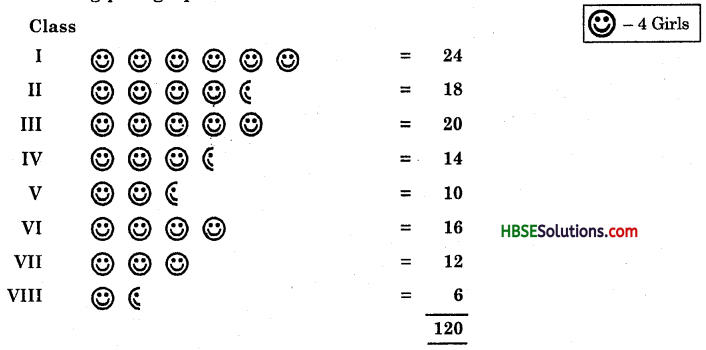Observe this pictograph and answer the following questions :
(a) Which class has the minimum number of girl students ?
(b) Is the number of girls in class VI less than the number of girls in class V ?
(c) How many girls are there in class VII ?
(d) What other inferences can you draw from this pictograph ?
Solution:
(a) Class VIII has the minimum number of girl students (6).
(b) No, the number of girl students in class VI are not less than the number of girls in class V.
(c) In class VII, there are 12 girl students.
(d) This pictograph shows that the maximum number of girl students (24) are in class I and the total number of girls in the school are 120.

Question 6.
In a village, six fruit merchants sold the following number of fruit baskets in a particular season :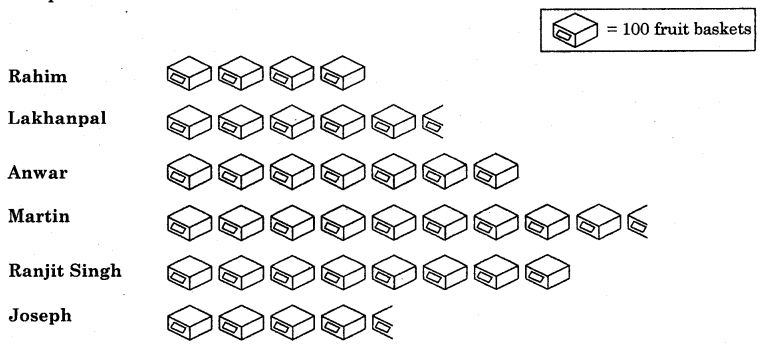Observe this pictograph and answer the following questions :
(a) Which merchant sold the maximum number of baskets ?
(b) How many fruit baskets were sold by Anwar ?
(c) The merchants who have sold 600 or more number of baskets are planning to buy a godown for the next season. Can you name them ?
Solution:
(a) Martin sold the maximum number of baskets.
(b) 700 fruit baskets were sold by Anwar.
(c) Anwar, Martin and Ranjit Singh.